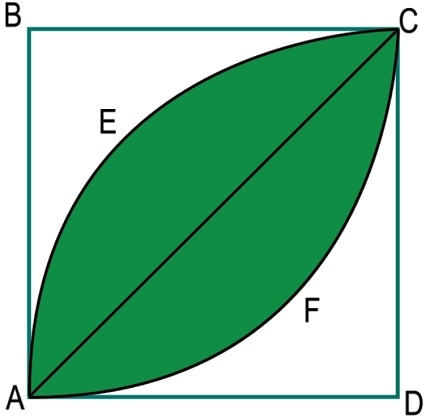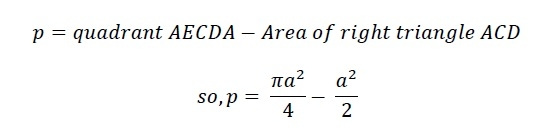# Area of a leaf inside a square?

CServer Side ProgrammingProgramming

Here we will see how to get the area of a leaf-like below, which is present inside the square ABCD. Each side of the square is of length ‘a’.The leaf has two equal parts. Area of each part is said p, now −And the area of the full leaf is 2p.

## Example

#include <iostream>
using namespace std;
float leafArea(float a){
return (a * a * (3.1415/2 - 1));
}
int main() {
float square_side = 7.0f;
cout << "Leaf Area: " << leafArea(square_side);
}

## Output

Leaf Area: 27.9667